#### You may also like### I'm Eight

Find a great variety of ways of asking questions which make 8.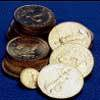### Five Coins

Ben has five coins in his pocket. How much money might he have?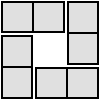### 4 Dom

Use these four dominoes to make a square that has the same number of dots on each side.

# Cubes

##### Age 7 to 11 Challenge Level:

In this activity we will be using cubes to make different arrangements. First, find three cubes that are all the same size and all have flat faces.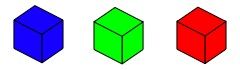Start by looking at just one of these cubes.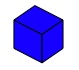Put it onto the table or another flat surface. How many square faces can you see? You are allowed to walk around the cube and bend down to see it, but you aren't allowed to pick the cube up. Click on the button below to check your answer:

There are five faces that we can see, because the sixth face is against the table.

Next, take two of the cubes. Put them down on the table, either separately or together. What different arrangements are there?

I've made these three arrangements with my cubes: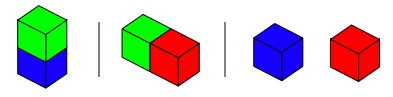How many square faces can I see in each case? Have a go at using your imagination to visualise how many I can see, and try making these arrangements yourself to check your answers. Then click on the button below:

In the first example, I can see nine faces - there are four lots of two faces around the sides, then one face at the top.

In the second example, I can see eight faces - one at each end, then three lots of two faces.

In the third example, I can see ten faces - there are two lots of five faces.

These three arrangements each showed me a different number of faces. Are there any other ways in which I could have arranged the cubes? Would these show me a different number of faces?

Think about how we can tell whether or not two arrangements are the same. For example, I could turn the second arrangement so that the red cube is pointing to the left instead - would this count as a different arrangement? Why/why not?

Finally, take all three of the cubes. Arrange these in some different ways. It might help to write or draw each arrangement to keep track of the ways that you have already tried. Here are some of my arrangements: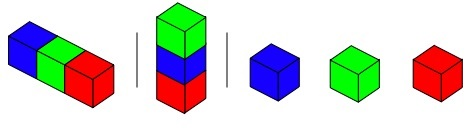How many square faces can I see in each of these three cases?

Now it's your turn! Use your three cubes every time, and see how many different ways there are to arrange them. How many faces can you see each time? Try to find all the possible answers.

#### Further challenge:

What happens if the faces don't line up completely? Look at the example below: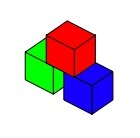I can see $14\frac{2}{3}$ faces on this arrangement. See if you can work out how I counted them, then click on the button below to check your answer:

On the red cube, I can see five whole faces, and if I bend down I can also see $\frac{1}{3}$ of a face underneath the red cube. On the blue cube, I can see four whole faces around the sides and then $\frac{2}{3}$ of a face on top. The green cube is the same as the blue cube, so this also shows me $4\frac{2}{3}$ faces. Adding all of these together gives me:
$5\frac{1}{3}$ + $4\frac{2}{3}$ + $4\frac{2}{3}$ = $14\frac{2}{3}$

How many arrangements can you find where the faces don't line up completely? How many different ways of doing this do you think there are?